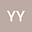loading page

Existence and nonexistence of global solutions for logarithmic hyperbolic equation
•## Abstract

In this paper, we study the initial-boundary value problem of a class of degenerate quasilinear hyperbolic equation with logarithmic nonlinearity. By applying Galerkin method and the logarithmic Sobolev inequality, we prove the existence of global weak solutions for this problem. Meanwhile,the global nonexistence of solutions is verified by means of the concavity analysis when the initial energy is positive and appropriately bounded.